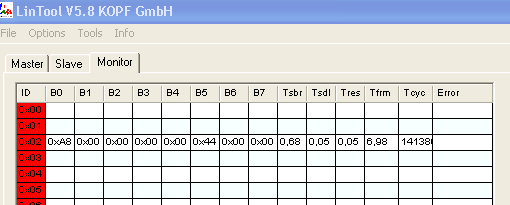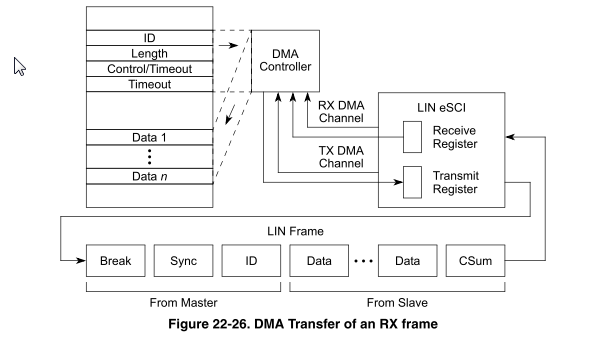## LIN/DMA transfer of a RX frame? (MPC55XX)

cancel
Showing results for
Did you mean:

## LIN/DMA transfer of a RX frame? (MPC55XX)

607 ViewsContributor II

Hi everybody,

I want to develop a LIN/DMA Transfer of RX frame. I tried to realize it but unfortunately without success. I cannot receive Data.

Has anyone worked on this before?

Regards,

3 Replies
263 ViewsContributor II263 ViewsContributor II

Hi Petr,

I tried to configure a lin and to generate a TX/LIN frame.
The frame is sent but the data were wrong.
)

Here is my code and a screenshot of what I sent (using LinTool).

/* Init eSCI B for LIN */
void initESCI_BLIN(/*baudrate*/)
{
//Enable LIN_EN
SIU.GPDO.B.PDO = 1;
/*The following setup applies for the LIN:*/
//The module is enabled by writing the ESCIB_CR2[MDIS]
ESCI_B.CR2.B.MDIS = 0;
//Both transmitter and receiver are enabled (ESCIB_CR1[TE] = 1; ESCIB_CR1[RE] = 1)
ESCI_B.CR1.B.TE = 1;
ESCI_B.CR1.B.RE = 1;
//The data format bit ESCIB_CR1[M], is set to 0 (8 data bits), and the parity is disabled (PE = 0)
ESCI_B.CR1.B.M = 0;
ESCI_B.CR1.B.PE = 0;
//ESCIB_CR1[TIE], ESCIB_CR1[TCIE], ESCIB_CR1[RIE] interrupt enable bits schould be used.
ESCI_B.CR1.B.TIE = 0;
ESCI_B.CR1.B.TCIE = 0;
ESCI_B.CR1.B.RIE = 0;
//Switch eSCI to lin mode (ESCIB_LCR[LIN] = 1)
ESCI_B.LCR.B.LIN = 1;
/*The LIN standart requires that the break character always be 13 bits long (ESCIB_CR2[BRK13] = 1).
the eSCI will work with BRK13 = 0. but it will violate LIN 2.0.*/
ESCI_B.CR2.B.BRK13 = 1;
/*Normally, bit errors should cause the LIN FSM to reset, stop driving the bus immediately, and
stop further DMA requests until the BERR flag has been cleared. Set ESCIx_LCR[LDBG] =
0, ESCIx_CR2[SBSTP] = 1, and ESCIx_CR2[BSTP] = 1 to accomplish these functions.*/
ESCI_B.LCR.B.LDBG = 0;
ESCI_B.CR2.B.SBSTP = 1;
ESCI_B.CR2.B.BSTP = 1;
/*Fast bit error detection provides superior error checking, so ESCIx_CR2[FBR] should be set;
normally it will be used with ESCIx_CR2[BESM13] = 1.*/
ESCI_B.CR2.B.BESM13 = 1;
/*The error indicators NF, FE, BERR, STO, PBERR, CERR, CKERR, and OVFL should be enabled.*/
ESCI_B.SR.B.NF = 1;
ESCI_B.SR.B.FE = 1;
ESCI_B.SR.B.STO = 1;
ESCI_B.SR.B.PBERR = 1;
ESCI_B.SR.B.CERR = 1;
ESCI_B.SR.B.CKERR = 1;
ESCI_B.SR.B.OVFL = 1;
ESCI_B.CR2.B.FBR = 1;
//Baud Rate value
ESCI_B.CR1.B.SBR = 0x01A1; // Baud Rate value = System Clock(128 MhH) / (16 * 19200)
/* Reset the internal transmitter controller using bit SCI_LCR[LRES] */
ESCI_B.LCR.B.LRES = 0;
ESCI_B.LCR.B.PRTY = 1;
/*Enable the transmit DMA feature */
ESCI_B.CR2.B.TXDMA = 1;
/*Enable the receive DMA feature */
ESCI_B.CR2.B.RXDMA = 1;

}

/*
* This function generates a LIN TX frame.

*uint8_t id = 0x2;
*uint8_t ch[] = {0x12, 0x25, 0x18, 0x19, 0x12, 0x14, 0x69, 0x15};
*uint8_t length = 8;
*LINTxFrame(id,length, &ch);
*/
void LINTxFrame(uint8_t id, uint8_t length, uint8_t *payload)
{
uint8_t DMATxFrame[length + 3];

/*Write LIN TX frame header and control information */
DMATxFrame = id;
DMATxFrame = length;
DMATxFrame = 0xD0; /* For LIN TX frame, TD must be set to 1 LIN 2.0*/

EDMA.TCD[DMA_CH].SSIZE = 0;
EDMA.TCD[DMA_CH].SOFF = 1;
EDMA.TCD[DMA_CH].SLAST = -(length + 3);
EDMA.TCD[DMA_CH].SMOD = 0;

EDMA.TCD[DMA_CH].DSIZE = 0;
EDMA.TCD[DMA_CH].DOFF = 0;
EDMA.TCD[DMA_CH].DLAST_SGA = 0;
EDMA.TCD[DMA_CH].DMOD = 0;

EDMA.TCD[DMA_CH].NBYTES = 1;
EDMA.TCD[DMA_CH].BITER = length + 3;
EDMA.TCD[DMA_CH].CITER = length + 3;
EDMA.TCD[DMA_CH].D_REQ = 1;
EDMA.TCD[DMA_CH].INT_HALF = 0;
EDMA.TCD[DMA_CH].INT_MAJ = 0;
EDMA.TCD[DMA_CH].E_SG = 0;
EDMA.TCD[DMA_CH].BWC = 0;
EDMA.TCD[DMA_CH].START = 0;
EDMA.TCD[DMA_CH].DONE = 0;
EDMA.TCD[DMA_CH].ACTIVE = 0;

EDMA.SERQR.R = DMA_CH; /* Enable EDMA channel */
}

263 ViewsNXP TechSupport

Hi,

as you mentioned MPC55xx, the eSCI is used and you want to do Master receiving using a DMA?

Two DMA channels can be used when executing an RX frame: one to transfer the header/control information from a memory location to the ESCIx_LTR, and one to transfer the incoming data bytes from the ESCIx_LRR to a table in memory.After the last byte from the RX frame has been stored, the DMA controller can indicate completion to the CPU.

BR, Petr